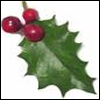#### You may also like### Giant Holly Leaf

Find the perimeter and area of a holly leaf that will not lie flat (it has negative curvature with 'circles' having circumference greater than 2πr).Given a square ABCD of sides 10 cm, and using the corners as centres, construct four quadrants with radius 10 cm each inside the square. The four arcs intersect at P, Q, R and S. Find the area enclosed by PQRS.### Get Cross

A white cross is placed symmetrically in a red disc with the central square of side length sqrt 2 and the arms of the cross of length 1 unit. What is the area of the disc still showing?

# Triangles and Petals

##### Age 14 to 16Challenge Level
Look at the equilateral triangle rotating around the equilateral triangle. It produces a flower with three petals:If each equilateral triangle has side length $r$, can you work out the perimeter of the flower's petals?

Now consider a flower made by the triangle rotating about a square with side length $r$ - what is the perimeter of the petals now?What is the perimeter when the centre of the flower is a regular pentagon, hexagon, heptagon...?
What can you say about the increase in perimeter as the number of sides of the centre shape increases?
Can you explain this increase?

What would be the perimeter of a flower whose centre is a regular $100$-sided polygon with side length $r$?

It may help to work in terms of $\pi$ throughout this problem.